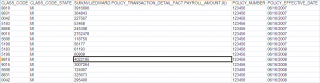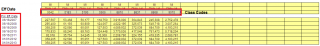[Solved] Attempting to sort from multiple arrays and input into Excel

Recommended Posts

I have been working on a project for an insurance customer to connect to an Oracle db and pull a bunch of policy holder info. They would then like the data placed into an Excel spreadsheet. Unfortunately, because the data sets that I need to work from will be different each time, I'm finding it difficult to fulfill the objectives.

1. I pull the data from the database and plug it into a temp excel spreadsheet, performing some minor formatting. The temp worksheet looks like this:2. I create an array of unique effective dates, and an array of unique class codes. Always 7 effective dates (not always the same dates), but anywhere from 1 to 16 Class Codes. These numbers are then plugged into the Master worksheet:3. I then need to iterate through each row in the temp worksheet. I need to plug the sum value into the correct cell, by both the class code and the effective date. I was able to create something that works very well (below), but only when I know the value and number of class codes:

\$b = 32
\$c = 32

For \$i = 2 To 500
\$ccode = _ExcelReadCell(\$oExcel, \$i, 1)
Select
Case \$ccode = ""
ExitLoop
Case \$ccode = "0042"
\$line = \$b
\$effDate = _ExcelReadCell(\$oExcel, \$i, 5)
\$sum = _ExcelReadCell(\$oExcel, \$i, 3)
_ExcelWriteCell(\$oExcel1, \$effDate, \$b, 1)
_ExcelWriteCell(\$oExcel1, \$sum, \$b, 3)
\$b += 1
Case \$ccode = 5183
\$line1 = \$b
\$effDate = _ExcelReadCell(\$oExcel, \$i, 5)
\$sum = _ExcelReadCell(\$oExcel, \$i, 3)
_ExcelWriteCell(\$oExcel1, \$effDate, \$c, 1)
_ExcelWriteCell(\$oExcel1, \$sum, \$c, 4)
\$c += 1
EndSelect
Next

_ExcelBookSaveAs(\$oExcel1, @DesktopDir & "\Output.xls", "xls", 0, 1)

I'm guessing I would need to do something like this, but have yet to get it to work:

Start with the array of class codes.

Loop through each row in the temp workbook.

On each row, find the effective date and jump to that row in the Master Workbook.

On each row, find the class code and jump to that column in the Master Workbook.

Write that row's SUM value to the corresponding cell in the Master Workbook.

Any suggestions would be greatly appreciated, or if there is another way to do this logically.

Edited by JLogan3o13

√-1 2^3 ∑ π, and it was delicious!

Share on other sites

Could you please modify your example so that the variables get meaningful names? Would help to understand your code.

If \$i is the linename then please name it \$iLine, the currently processed column should be named \$iColumn etc.

Could save a lot of time to help you.

My UDFs and Tutorials:

Spoiler

UDFs:
Active Directory (NEW 2019-08-19 - Version 1.4.13.0) - Download - General Help & Support - Example Scripts - Wiki
OutlookEX (NEW 2019-07-24 - Version 1.3.6.0) - Download - General Help & Support - Example Scripts - Wiki
Outlook Tools (NEW 2019-07-22 - Version 0.6.0.0) - Download - General Help & Support - Wiki
ExcelChart (2017-07-21 - Version 0.4.0.1) - Download - General Help & Support - Example Scripts
PowerPoint (2017-06-06 - Version 0.0.5.0) - Download - General Help & Support
Excel - Example Scripts - Wiki
Word - Wiki
Taks Scheduler (NEW 2019-10-09 - Version 0.9.0.0) - Download - General Help & Support - Wiki

Tutorials:

Share on other sites

Thanks for the suggestion, I went through and tidied the code a bit. I believe I have resolved the issue as well, though I am always open to suggestions for improvement (maybe a better way to get the index rather than going back for it each time). Below is the current, working solution:

Gather the list of Effective Dates:

Func _writeEffectiveDates()

\$rowCount = \$oExcel.Cells.CurrentRegion.Rows.Count

\$aTempArray = _ExcelReadArray(\$oExcel, 1, 5, \$rowCount, 1)
_ArrayDelete(\$aTempArray, 0)
Global \$aDates = _ArrayUnique(\$aTempArray)

\$iColumn = 1 ;Begin inserting data in the first column on the Master workbook.
\$iRow = 32   ;Begin inserting date on this row on the Master workbook.

For \$i = 1 To \$aDates
_ExcelWriteCell(\$oExcel1, \$aDates[\$i], \$iRow, \$iColumn)
\$iRow += 1
Next

EndFunc

Then gather the list of CLASS CODES:

\$rowCount = \$oExcel.Cells.CurrentRegion.Rows.Count

\$aTempArray = _ExcelReadArray(\$oExcel, 1, 1, \$rowCount, 1)
_ArrayDelete(\$aTempArray, 0)
Global \$aCCodes = _ArrayUnique(\$aTempArray)
_ArraySort(\$aCCodes, 0, 1)

\$iColumn = 3 ;Begin inserting data in the first column on the Master workbook.
\$iRow = 30   ;Begin inserting date on this row on the Master workbook.

For \$i = 1 To \$aCCodes
_ExcelWriteCell(\$oExcel1, \$aCCodes[\$i], \$iRow, \$iColumn)
\$iColumn +=1
Next

Finally, find the row and column the SUM value needs to go into, based on the Effective Date (row) and CLASS CODE (column)

\$rowCount = \$oExcel.Cells.CurrentRegion.Rows.Count
\$iCCColumn = 1
\$iSumColumn = 3
\$iEffColumn = 5
\$iStartRow = 31
\$iStartColumn = 2

For \$i = 2 To \$rowCount
\$cCode = _ExcelReadCell(\$oExcel, \$i, \$iCCColumn)
\$sum = _ExcelReadCell(\$oExcel, \$i, \$iSumColumn)
\$effDate = _ExcelReadCell(\$oExcel, \$i, \$iEffColumn)
\$iIndex1 = _ArraySearch(\$aDates, \$effDate, 0, 0, 0, 1)
\$iIndex2 = _ArraySearch(\$aCCodes, \$cCode, 0, 0, 0, 1)
_ExcelWriteCell(\$oExcel1, \$sum, \$iStartRow + \$iIndex1, \$iStartColumn + \$iIndex2)
Next

√-1 2^3 ∑ π, and it was delicious!

Create an account or sign in to comment

You need to be a member in order to leave a comment

Create an account

Sign up for a new account in our community. It's easy!

Register a new account